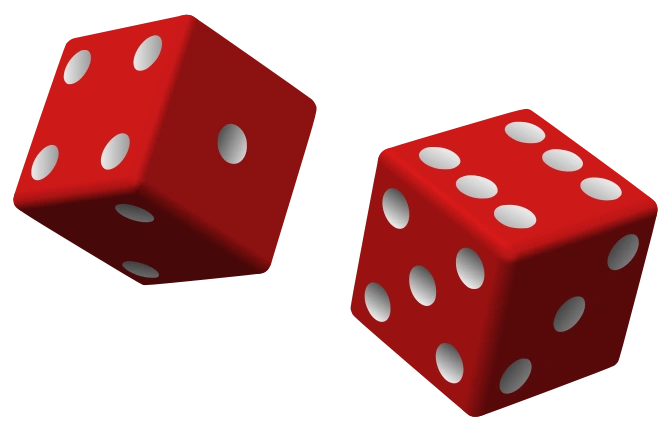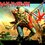# Stochastic Programming - I (Interpreting basic probabilistic results)

This is my first Computer Science note so I would love a bit of feed back in the comments.img1

$$\textbf{A Probabilistic World:}$$

The most comforting thing about Newtonian Mechanics is that everything happens for a reason.For example,if you push one end of the lever,the other end goes up.You throw an object and it travels in a parabolic path.The physical world is a completely deterministic place-all the future states are derived form the previous s ones.For centuries we had this prevailing scientific wisdom,then came the Copenhagen doctrine,led by Bohr and Heisenberg.The proponents of this doctrine argued that at the most fundamental level,the behavior of a physical system cannot be determined.This led to a serious debate regarding the validity of causal non-determinism,ie every event is not caused by previous events.People like Einstein and Schrodinger found this philosophy unacceptable as exemplified by Einstein's often repeated comment - "God does not play dice".The question of causal non-determinism is still unsettled but there is ample evidence to prove that certain systems can only be modeled accurately by stochastic processes.A process is called stochastic if it's next step depends on both previous states and some random event.

$\textbf{Interpreting basic results:}$

We all know how to calculate the basic probability of occurrence of certain events.But how do we interpret the significance of the results obtained?The answer is in this age of fast computers we can run multiple simulations of an event and record the outcomes and compare the results with the mathematically calculated value!Then in front of our own eyes we can see how powerful the theory of probability is!img2

$\textbf{The Story of Blaise Pascal:}$

We all know that one of the most influential figures in the history of Probability Theory was Blaise Pascal.His interest in the field began when a friend asked him the following question

"Would it be profitable given 24 rolls of a pair of fair dice to bet against there being at least one double six"

Pascal and Fermat exchanged a number of letters to solve this issue which apparently looks easy in the 21st century.Let us calculate it.

The probability of rolling $6$ on one dice = $\frac{1}{6}$

The probability of rolling $6$ on both dice = $\frac{1}{6^{2}}$ = $\frac{1}{36}$

The probability of not rolling a double $6$ on first roll = $(1 - \frac{1}{36})$ = $\frac{35}{36}$

The probability of not rolling a double $6$ on 24 rolls = $(\frac{35}{36})^{24}$ = $0.508596123869$.img3

$\textbf{The Law of Large Numbers:}$

It states that in repeated independent tests,with actual probability $p$ of a particular outcome in each test,the change that the fraction of times that outcome occurs differs from $p$ converges to $0$ as the number of trails go to infinity.img4

In other words we are going to organize a large number of trails of Pascal's experiment and expect that the simulation results will get very close to the mathematically calculated value.

$\textbf{Constructing a Stochastic Program:}$

The programming language we are going to use is Python.We will use Python's random module to construct a program to check the results.It is given by the following code with the comments explaining each line.

  1 2 3 4 5 6 7 8 9 10 11 12 13 14 15 16 17 18 import random def rollDie(): """returns a random int between 1 and 6""" return random.choice([1,2,3,4,5,6]) def checkPascal(numTrials,roll): yes = 0.0 #collector variable for favorable outcomes for i in range(numTrials): #executing trials for j in range(24): #rolling the pair 24 times d1 = roll() #rolling the first dice d2 = roll() #rolling the second dice if d1 == 6 and d2 == 6:#checking favorable outcome yes += 1 #increasing the collector variable break print "Probability of losing",1 - (yes/numTrials) checkPascal(10000,rollDie) # running the simulation 10000 times 

When I ran the program, the outcome was $0.5067892$.Pretty close to Pascal's math!Try it out in a Python 2.7 interpreter!!

$\textbf{Footnotes:}$

This note was inspired by a chapter from "Introduction to Computaion and programming in Python" by John V.Guttag.

Those of you wondering about the matrix picture it is because the movie depicts the ultimate computer simulation.img57 years, 2 months ago

This discussion board is a place to discuss our Daily Challenges and the math and science related to those challenges. Explanations are more than just a solution — they should explain the steps and thinking strategies that you used to obtain the solution. Comments should further the discussion of math and science.

When posting on Brilliant:

• Use the emojis to react to an explanation, whether you're congratulating a job well done , or just really confused .
• Ask specific questions about the challenge or the steps in somebody's explanation. Well-posed questions can add a lot to the discussion, but posting "I don't understand!" doesn't help anyone.
• Try to contribute something new to the discussion, whether it is an extension, generalization or other idea related to the challenge.

MarkdownAppears as
*italics* or _italics_ italics
**bold** or __bold__ bold
- bulleted- list
• bulleted
• list
1. numbered2. list
1. numbered
2. list
Note: you must add a full line of space before and after lists for them to show up correctly
paragraph 1paragraph 2

paragraph 1

paragraph 2

[example link](https://brilliant.org)example link
> This is a quote
This is a quote
    # I indented these lines
# 4 spaces, and now they show
# up as a code block.

print "hello world"
# I indented these lines
# 4 spaces, and now they show
# up as a code block.

print "hello world"
MathAppears as
Remember to wrap math in $$ ... $$ or $ ... $ to ensure proper formatting.
2 \times 3 $2 \times 3$
2^{34} $2^{34}$
a_{i-1} $a_{i-1}$
\frac{2}{3} $\frac{2}{3}$
\sqrt{2} $\sqrt{2}$
\sum_{i=1}^3 $\sum_{i=1}^3$
\sin \theta $\sin \theta$
\boxed{123} $\boxed{123}$

Sort by:

Eddie, nice note. One thing tangential to your main thrust, but still important: you should be very careful about the notion that "events aren't caused by previous events". Even in quantum mechanics, if you know the state of the system at some time $t=0$ the state at any future time can be explicitly calculated. Hence QM is also thoroughly deterministic in this sense. The Copenhagen interpretation, in the popular lingo that randomly one particular value is picked when a measurement occurs, has gone through a lot of adjustment in the last few decades and we now have a much better idea as to how this can actually happen in a manner that respects the deterministic nature of quantum mechanics. If you want to explore, read a little more about decoherence.

Staff - 7 years, 2 months ago

Thank you for your comment Sir.I read up on decoherence. But can Heisenberg's uncertainty principle also be interpreted deterministically??

- 7 years, 2 months ago

What are the values that you want to be precisely determined and evolved deterministically? position and momentum?

Staff - 7 years, 2 months ago

Yes sir,that's what I meant........

- 7 years, 2 months ago

those are measurements on the fundamentally evolving object. Requiring that position and momentum are always determined and evolving according to some rule is not a correct belief about nature.

Staff - 7 years, 2 months ago

I do love the pic, and nice code to replicate Pascal.

- 7 years, 2 months ago

I always like to see code examples. Helps me learn Python better!

I ran it 6 times and got results ranging from .5036 to .5138, but I did need to change the indentation of the print statement. Maybe this will help others that try it out.

Thanks for the interesting discussion!

- 7 years ago

luv what u have written

- 7 years, 2 months ago

Eddie i really love your notes and pics but may you plz tell me smthng about fermat's no. theory..... (Y)

- 7 years, 1 month ago

Fermat made many significant contributions to the theory of numbers the most famous of which is Fermat's Last theorem....which one do u mean?

- 7 years, 1 month ago